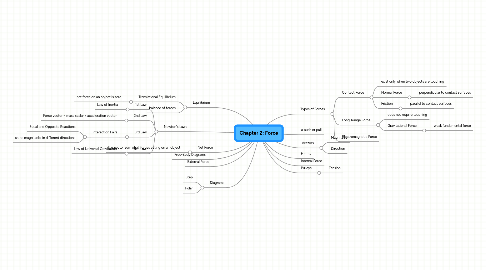# Chapter 2: Force

Get Started. It's FreeChapter 2: Force## 1. Newton's Laws

### 1.1. 1st Law

1.1.1. Law of Inertia

### 1.2. 2nd Law

1.2.1. Force vector = mass scalar x acceleration vector

### 1.3. 3rd Law

1.3.1. Interaction Pairs

1.3.1.1. Equal and Opposite Reactions

1.3.1.2. same magnitude, in different directions

### 1.4. 4th Law

1.4.1. Law of Universal Gravitation

## 5. Types of Forces

### 5.1. Contact Force

5.1.1. exist only when two objects are touching

5.1.2. Normal Force

5.1.2.1. perpendicular to contact surfaces

5.1.3. Friction

5.1.3.1. parallel to contact surfaces

### 5.2. Long-Range Force

5.2.1. does not require touching

5.2.2. Gravitational Force

5.2.2.1. weak fundamental force

## 8. Equilibrium

### 8.1. Translational Equilibrium

8.1.1. net force on an object is zero